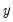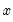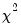Object Reference : Graph Creation Commands : Optional Graph Components

Optional Graph Components
The following sections describe optional components that may be used as part of a graph specification:
A categorical spec may be added to most graph commands to create a categorical graph.
An auxiliary spec may be added to an XY graph command ( scat, scatmat, scatpair, xyarea, xybar, xyline, xypair) to add fit lines (or confidence ellipses) to the graph.
Categorical Spec
Adding a categorical spec to a graph commands produces a categorical graph. For example, adding a categorical spec to a bar graph generates a categorical bar graph using the factors defined by the spec; adding a categorical spec to an XY-line graph creates a categorical XY-line graph.
The categorical spec is used to specify the factors used in categorization. It may include one or more within and across factors of the following form:
within(factor_name[, factor_options])
or
across(factor_name[, factor_options])
where factor_name is the name of a series used to define a category along with the factor_options. Multiple factors of a given type should be listed in order from most slowly to fastest varying.
Categorical graphs are not supported for matrix object views. Note also that use of a categorical specification will override any panel options.
Factor options

 incna include NA category inctot include total category iscale, cscale individua/common scale for this factor.The default is individual for the “@series” factor, and common for all others. iscalex, cscalex individual/common X axis scale for this factor.The default is individual for the “@series” factor, and common for all others. iscaley, cscalex individual/common Y axis scale for this factor.The default is individual for the “@series” factor, and common for all others. bintype=type bin type, where type can be: “auto” (default), “quant” - quantile binning, “value” - value binning, “none” - forces no binning. bincount=int int is the number of quantile bins or maximum number of value bins. dispname use display name in labels label=key key can be: “auto” (default), “value” - factor value only, “both” - factor name and value. ncase=key sets the capitalization for factor names in labels, where key can be: “upper”, “lower”, “title”. The default is to preserve case. vcase=key sets the capitalization for factor values in labels, where key can be: “upper”, “lower”, “title”. The default is to preserve case.
Categorical spec examples
profit.boxplot across(firm)
displays a categorical boxplot graph of PROFITS using distinct values of FIRM to define the categories, and displaying the graphs in multiple frames.
profit.boxplot across(firm, dispname, iscale)
shows the same graph with individual scaling for each of the frames, using the displayname in labels.
profit.boxplot within(firm, inctot, label=value)
displays a boxplot graph categorized by firm (with an added category for the total), with all of the graphs in a single frame and the category value used as labels.
ser1.bar(contract=sum) across(firm, dispname) within(income, bintype=quant, bincount=4)
constructs a categorical bar graph of the sum of SER1 values within a category. Different firms are displayed in different graph frames, using the display name as labels, with each frame containing bars depicting the sum of SER1 for each income quartiles.
ser1.bar(contract=mean, elemcommon=1) within(sex) within(union)
creates a bar graph of mean values of within categories based on both SEX and UNION. Categories for the distinct elements of UNION will be depicted using different bar colors, with the color assignment repeated for different values of SEX.
By default, the multiple series in a group are treated as the first (most slowly varying) across factor. To control the treatment of this implicit factor, you may use the “@series” keyword in a within or across specification; if the factor is not the first one of its type listed, it will be treated as the last factor. Thus:
g1.boxplot within(sex) within(union)
creates an boxplot for within categories based on both SEX and UNION. Since we have not specified behavior for the implicit series factor in GRP1, the series in the group will be treated as the first across factor and will be displayed in a separate frame.
g1.qqplot theory within(age)
displays theoretical qq-plots with the series in G1 treated as the within factor and @SERIES treated as the across factor. The qq-plots for each series in G1 will be displayed in separate frames, with multiple qq-plots for each AGE category shown in each frame.
g1.distplot hist kernel across(sex) across(@series) across(age)
displays histograms and kernel density plots where the implicit factor is the last across factor.
group mygrp oldsales newsales
mygrp.bar(contract=min) within(@series) within(age)
displays bar graphs of the minimum values for categories defined by distinct values of AGE (and the two series). All of the bars will be displayed in a single frame with the bars for OLDSALES grouped together followed by the bars for NEWSALES.
mygrp.bar(contract=median, elemcommon=2) across(firm) across(@series) across(age)
also adds an additional categorization using the FIRM identifiers. The observations for a given firm are grouped together. Within a firm, the bars for the OLDSALES and NEWSALES, which will be depicted using different colors, will be grouped within each age category. The color assignment to OLDSALES and NEWSALES will be repeated across firms and ages (note that @SERIES is treated as the last across factor).
Auxiliary Spec
You may add one or more fit lines or confidence ellipses to your XY graph using an auxiliary spec. (Note that auxiliary specs are not allowed with stacked XY graphs.)
For a description of the available fit line types, see “Auxiliary Graph Types”.
The auxiliary spec should be in the form:
fitline_type(type_options)
where fitline_type is one of the following keywords:

 linefit Add a regression line. kernfit Add a kernel fit line. nnfit Add a nearest neighbor (local) fit line. orthreg Add an orthogonal regression line. cellipse Add a confidence ellipse. user Add a user-specified line.
Each fit line type has its own set of options, to be entered in type_options:
To save the data from selected auxiliary graph types in the workfile, see Group::distdata.
Linefit Options

 yl Take the natural log of first series or column,. yi Take the inverse of. yp=number Take y to the power of the specified number. yb=number Take the Box-Cox transformation ofwith the specified parameter. xl Take the natural log of. xi Take the inverse of. xp=number Taketo the power of the specified number. xb=number Take the Box-Cox transformation ofwith the specified parameter. xd=integer Fit a polynomial ofup to the specified power. m=integer Set number of robustness iterations. leg=arg Specify the legend display settings, where arg can be: “def” - default, “n” - none, “s” - short, “det”- detailed.
If the polynomial degree ofleads to singularities in the regression, EViews will automatically drop high order terms to avoid collinearity.
Linefit Examples
group g1 x y z w
g1.scatpair linefit(yl,xl)
displays a scatterplot of Y against X and W against Z, together with the fitted values from a regression of log Y on log X and log W on log Z.
g1.scat linefit linefit(yb=0.5,m=10)
shows scatterplots of Y, Z, and W along the vertical axis and X along the horizontal axis, and superimposes both a simple linear regression fit and a fit of the Box-Cox transformation of the vertical axis variable against X, with 10 iterations of bisquare weights,.
Kernfit Options

 k=arg (default=“e”) Kernel type: “e” (Epanechnikov), “r” (Triangular), “u” (Uniform), “n” (Normal–Gaussian), “b” (Biweight–Quartic), “t” (Triweight), “c” (Cosinus). b=number Specify a number for the bandwidth. b Bracket bandwidth. ngrid=integer (default=100) Number of grid points to evaluate. x Exact evaluation of the polynomial fit. d=integer (default=1) Degree of polynomial to fit. Set “d=0” for Nadaraya-Watson regression. leg = arg Specify the legend display settings, where arg can be: “def” - default, “n” - none, “s” - short, “det”- detailed.
Kernfit Examples
group gg weight height length volume
gg.scat kernfit kernfit(d=2, b)
displays scatterplots with HEIGHT, LENGTH, and VOLUME on the vertical axis and WEIGHT on the horizontal axis, along with the default kernel regression fit, and a second-degree polynomial fit with bracketed bandwidths.
gg.scatmat kernfit(ngrid=200)
displays a scatterplot matrix of the series in GG and fits a kernel regression of the Y-axis variable on the X-axis variable using 200 grid points.
Nnfit Options

 d=integer (default=1) Degree of polynomial to fit. b=fraction (default=0.3) Bandwidth as a fraction of the total sample. The larger the fraction, the smoother the fit. b Bracket bandwidth span. s Symmetric neighbors. Default is nearest neighbors. u No local weighting. Default is local weighting using tricube weights. m=integer Set number of robustness iterations. x Exact (full) sampling. Default is Cleveland subsampling. neval=integer (default=100) Approximate number of data points at which to compute the fit (if performing Cleveland subsampling). leg=arg Specify the legend display settings, where arg can be: “def” - default, “n” - none, “s” - short, “det”- detailed.
Nnfit Examples
group gr1 gdp90 cons90 gdp70 cons70
gr1.scatpair nnfit(x,m=3)
displays the nearest neighbor fit of CONS90 on GDP90 and of CONS70 on GDP70 with exact (full) sampling and 3 robustness iterations. Each local regression fits the default linear regression, with tricube weighting and a bandwidth of span 0.3.
gr1.scatpair nnfit nnfit(neval=50,d=2,m=3)
computes both the default nearest neighbor fit and a custom fit that fits a quadratic at 50 data points, using tricube robustness weights with 3 robustness iterations.
Orthreg Options

 leg=arg Specify the legend display settings, where arg can be: “def” - default, “n” - none, “s” - short, “det”- detailed.
Orthreg Examples
group gg weight height length volume
gro1.scatmat(l) orthreg
displays the orthogonal regression fit for each pair in the lower-triangle scatterplot matrix.
Cellipse Options

 size=arg Specify the confidence levels. c Usedistribution to compute the confidence ellipses. The default is to use the F-distribution. leg=arg Specify the legend display settings, where arg can be: “def” - default, “n” - none, “s” - short, “det”- detailed.
Cellipse Examples
group gro1 age income cons taxes
gro1.scat cellipse
displays the 95% confidence ellipse around the means of the plots of INCOME, CONS, and TAXES against AGE.
gro1.scat cellipse(size=0.95 0.85 0.75)
displays the 95%, 85%, and 75% confidence ellipses, computed using the chi-square distribution
vector(3) sizes
sizes.fill 0.95, 0.85, 0.75
gro1.scat cellipse(size=sizes)
displays the same graph.
User Options
A user specified line can be specified either using a pair of data points (where you specify the X and Y values for each point, or using a simple line specification with a Y-intercept, slope and, optionally, transformation value. Entering data point values overrides and simple line options.

 x1=arg Set the X (horizontal) value for the first data point. y1=arg Set the Y (vertical) value for the first data point. x2=arg Set the X (horizontal) value for the second data point. y2=arg Set the Y (vertical) value for the second data point. icept=arg Set simple line Y-intercept of value. Default is 0 slope=arg Set simple line slope. Default is 0. xl Use a logarithmic transformation on the X series. yl Use a logarithmic transformation on the Y series. xi Use an inverse transformation on the X series. yi Use an inverse transformation on the Y series. xp=arg Use a power transformation, with power equal to arg on the X series. yp=arg Use a power transformation, with power equal to arg on the Y series. xb=arg Use a Box-Cox transformation of order arg on the X series. yb=arg Use a Box-Cox transformation of order arg on the Y series. xd=arg Use a polynomial transformation of order arg on the X series.
User Examples
group gro1 age income
gro1.scat user(x1=3, y1=4, x2=10, y2=15)
Draws a user specified straight line joining the two points (4,3) and (15,10).
gro1.scat user(icept=5, slope=0.5, xp=2)
Draws a user specified line with an intercept of 5, a slope of 0.5 and a power transformation on the X series.